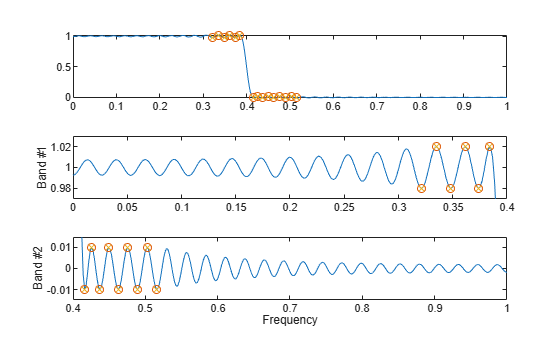# fircls

Constrained-least-squares FIR multiband filter design

## Syntax

``b = fircls(n,f,amp,up,lo)``
``fircls(n,f,amp,up,lo,"design_flag")``

## Description

example

````b = fircls(n,f,amp,up,lo)` generates a length `n` + 1 linear phase FIR filter. The frequency-magnitude characteristics of this filter match those given by vectors `f` and `amp`.```
````fircls(n,f,amp,up,lo,"design_flag")` enables you to specify visual display options for the filter design.```

## Examples

collapse all

Design a 150th-order lowpass filter with a normalized cutoff frequency of $0.4\pi$ rad/sample. Specify a maximum absolute error of 0.02 in the passband and 0.01 in the stopband. Display the design error and magnitude responses of the filter.

```n = 150; f = [0 0.4 1]; a = [1 0]; up = [1.02 0.01]; lo = [0.98 -0.01]; b = fircls(n,f,a,up,lo,"both");```
``` Bound Violation = 0.0788344298966 Bound Violation = 0.0096137744998 Bound Violation = 0.0005681345753 Bound Violation = 0.0000051519942 Bound Violation = 0.0000000348656 `````` Bound Violation = 0.0000000006231 ```

The Bound Violations denote the iterations of the procedure as the design converges. Display the magnitude response of the filter.

`fvtool(b)`## Input Arguments

collapse all

Filter order, specified as a real positive scalar.

Note

The `fircls` function always uses an even filter order for configurations with a passband at the Nyquist frequency (that is, highpass and bandstop filters). This is because for odd orders, the frequency response at the Nyquist frequency is necessarily 0. If you specify an odd-valued `n`, `fircls` increments it by 1.

Normalized frequency points, specified as a real-valued vector. The transition frequencies are in the range [0, 1], where 1 corresponds to the Nyquist frequency. The first point of `f` must be `0` and the last point must be `1`. The frequencies must be in increasing order.

Piecewise-constant desired amplitude of the frequency response, specified as a real-valued vector. The length of `amp` is equal to the number of bands in the response, `length(f)-1`.

Upper bounds for the frequency response in each band, specified as a real-valued vector with the same length as `amp`.

Lower bounds for the frequency response in each band, specified as a real-valued vector with the same length as `amp`.

Note

Normally, the lower value in the stopband is specified as negative. By setting `lo` equal to `0` in the stopbands, a nonnegative frequency response amplitude is obtained. Such filters are spectrally factored to obtain minimum phase filters.

Filter design display, specified as one of these:

• `"trace"` — View a textual display of the design error at each iteration step.

• `"plots"` — View a collection of plots showing the full-band magnitude response of the filter and a zoomed view of the magnitude response in each sub-band. All plots are updated at each iteration step. The O's on the plot are the estimated extremals of the new iteration and the X's are the estimated extremals of the previous iteration, where the extremals are the peaks (maximum and minimum) of the filter ripples. Only ripples that have a corresponding O and X are made equal.

• `"both"` — View both a textual display and plots.

## Output Arguments

collapse all

Filter coefficients, returned as a row vector of length `n` + 1.

## Algorithms

The `fircls` function uses an iterative least-squares algorithm to obtain an equiripple response. The algorithm is a multiple exchange algorithm that uses Lagrange multipliers and Kuhn-Tucker conditions on each iteration.

 Selesnick, I. W., M. Lang, and C. S. Burrus. “Constrained Least Square Design of FIR Filters without Specified Transition Bands.” Proceedings of the 1995 International Conference on Acoustics, Speech, and Signal Processing. Vol. 2, 1995, pp. 1260–1263.

 Selesnick, I. W., M. Lang, and C. S. Burrus. “Constrained Least Square Design of FIR Filters without Specified Transition Bands.” IEEE® Transactions on Signal Processing. Vol. 44, Number 8, 1996, pp. 1879–1892.